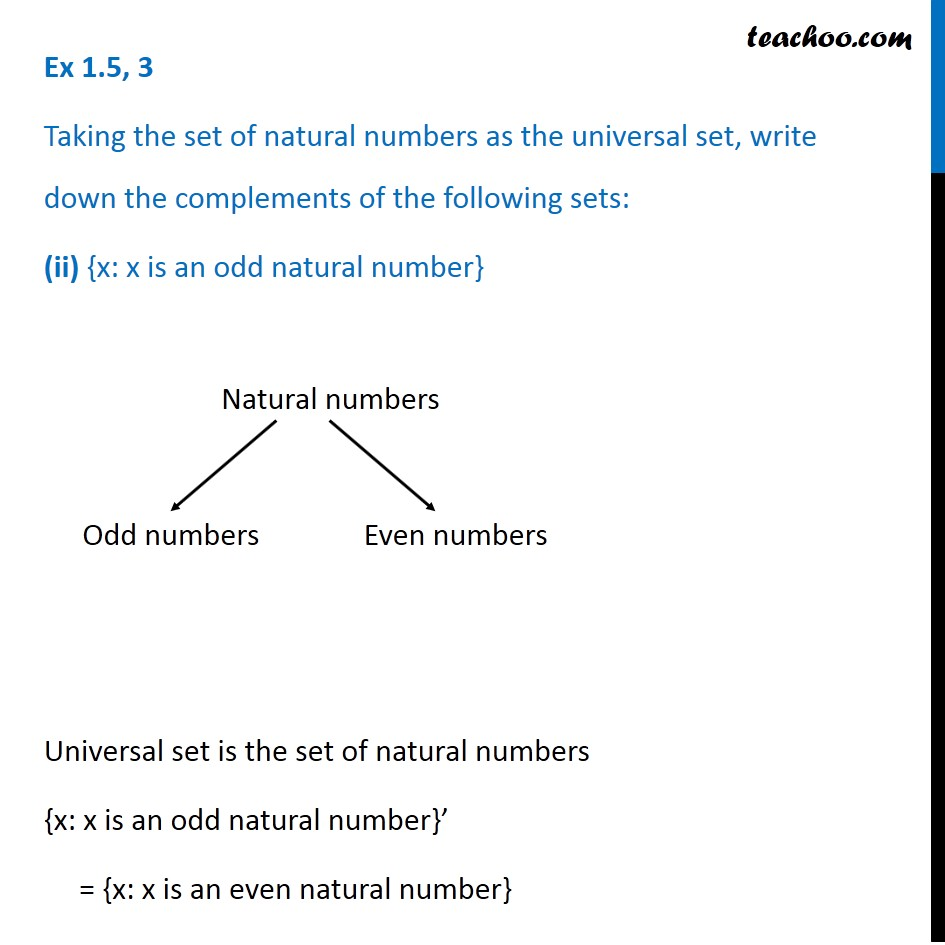1. Chapter 1 Class 11 Sets (Term 1)
2. Serial order wise
3. Ex 1.5

Transcript

Ex 1.5, 3 Taking the set of natural numbers as the universal set, write down the complements of the following sets: (ii) {x: x is an odd natural number} Universal set is the set of natural numbers {x: x is an odd natural number}’ = {x: x is an even natural number}